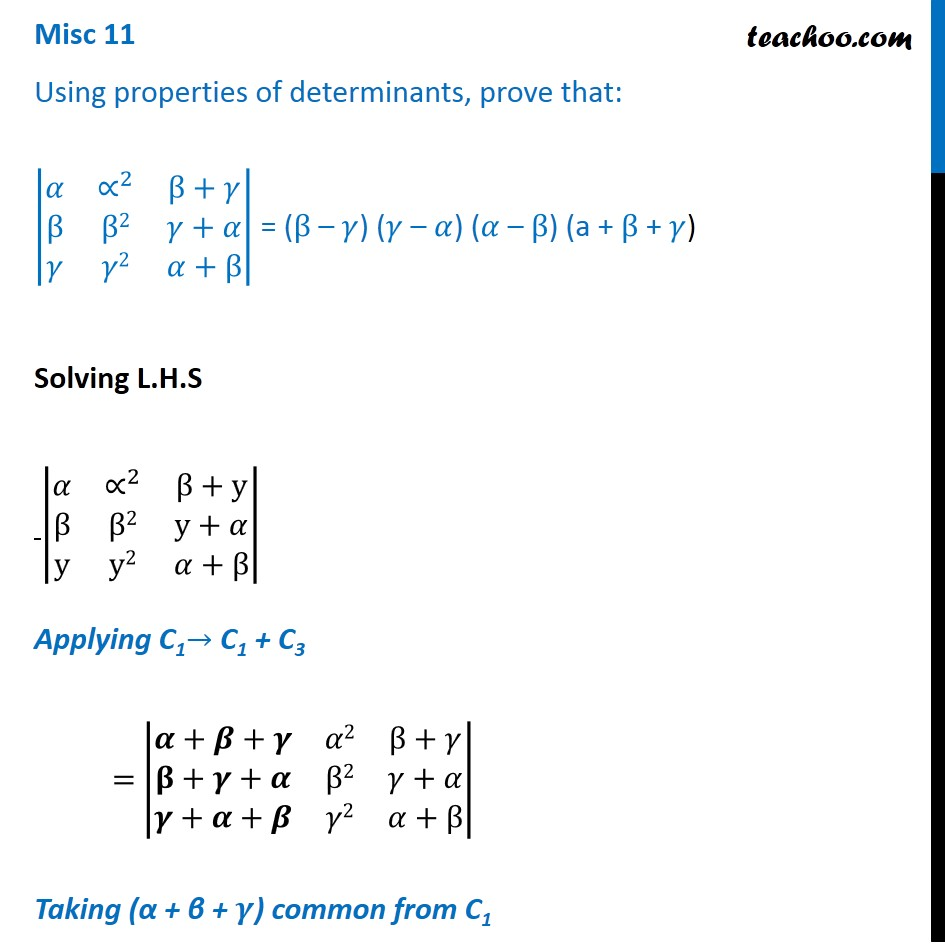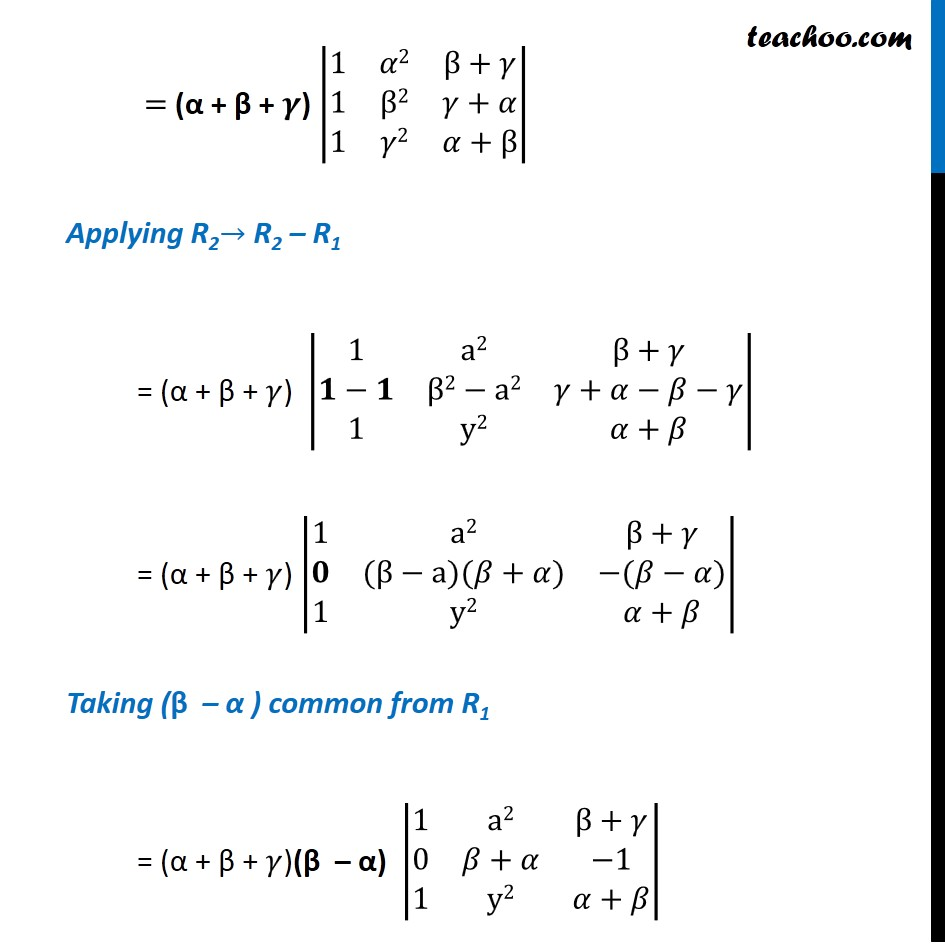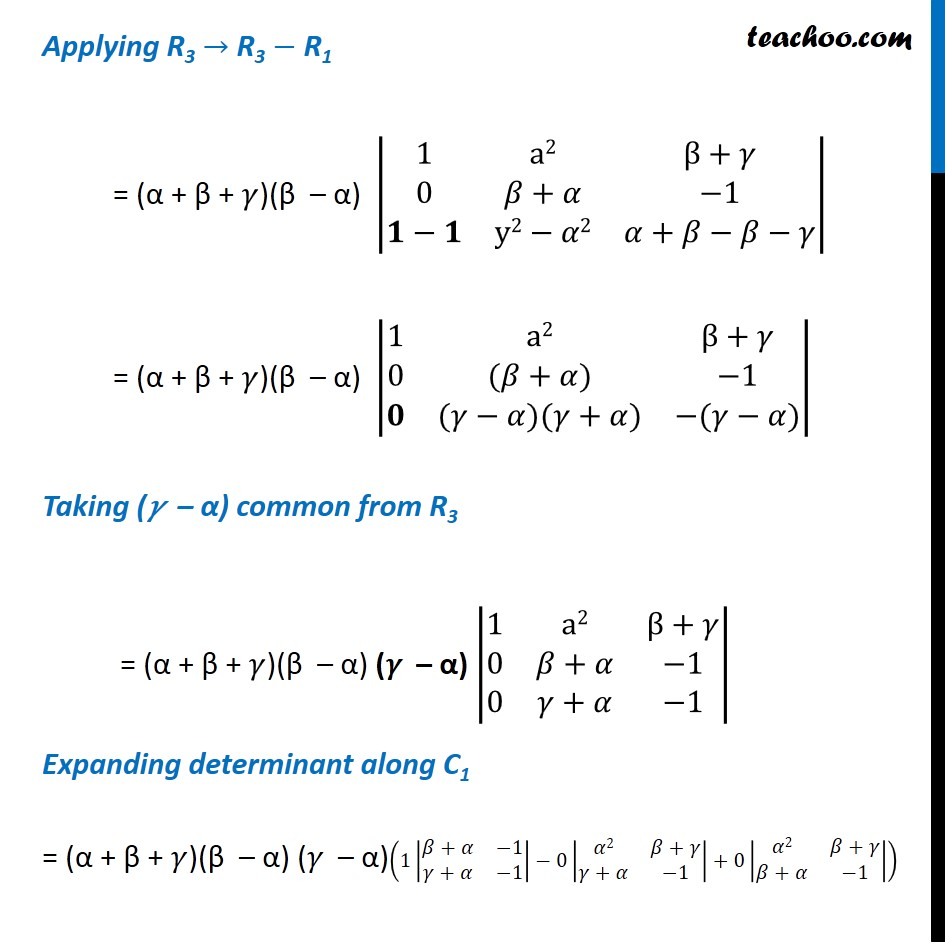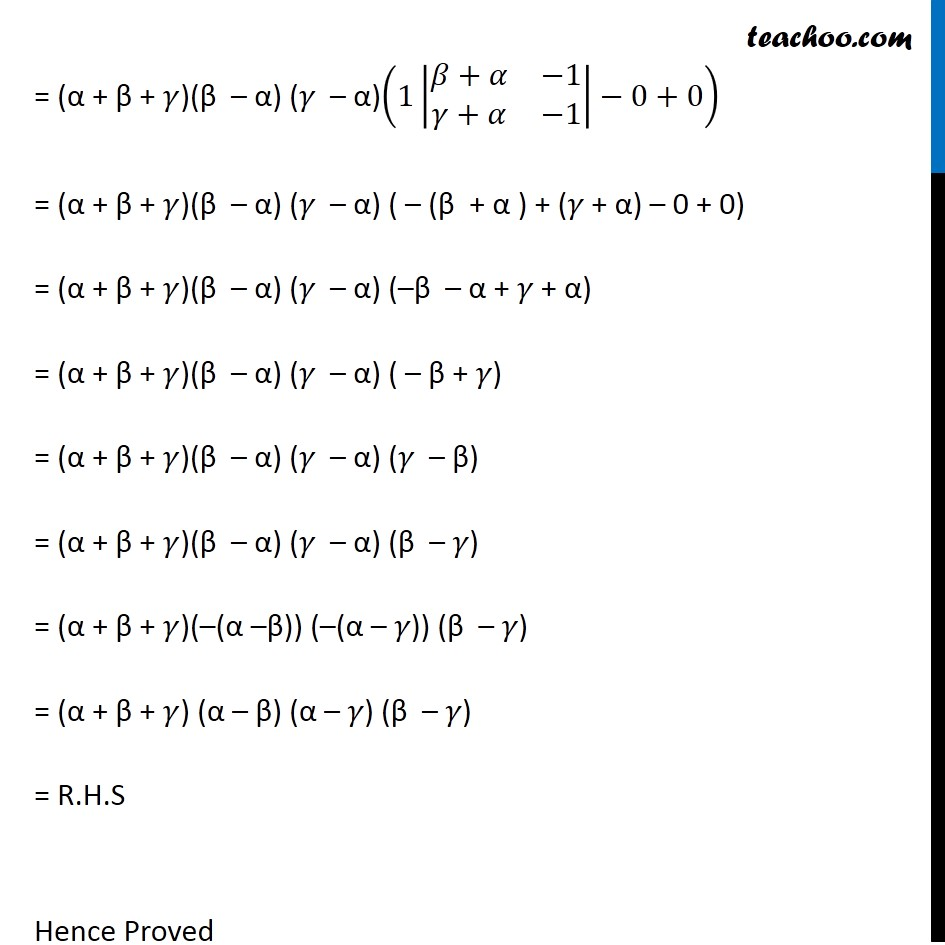Miscellaneous

Chapter 4 Class 12 Determinants
Serial order wiseLearn in your speed, with individual attention - Teachoo Maths 1-on-1 Class

### Transcript

Question 5 Using properties of determinants, prove that: |■8(𝛼&∝^2&β+𝛾@β&β2&𝛾+𝛼@𝛾&𝛾2&𝛼+β)| = (β – 𝛾) (𝛾 – 𝛼) (𝛼 – β) (a + β + 𝛾) Solving L.H.S |■8(𝛼&∝^2&β+y@β&β2&y+𝛼@y&y2&𝛼+β)| Applying C1→ C1 + C3 = |■8(𝜶+𝜷+𝜸&𝛼2&β+𝛾@𝛃+𝜸+𝜶&β2&𝛾+𝛼@𝜸+𝜶+𝜷&𝛾2&𝛼+β)| Taking (α + β + 𝜸) common from C1 = (α + β + 𝜸) |■8(1&𝛼2&β+𝛾@1&β2&𝛾+𝛼@1&𝛾2&𝛼+β)| Applying R2→ R2 – R1 = (α + β + 𝛾) |■8(1&a2&β+𝛾@𝟏−𝟏&β2−a2&𝛾+𝛼−𝛽−𝛾@1&y2&𝛼+𝛽)| = (α + β + 𝛾) |■8(1&a2&β+𝛾@𝟎&(β−a)(𝛽+𝛼)&−(𝛽−𝛼)@1&y2&𝛼+𝛽)| Taking (β – α ) common from R1 = (α + β + 𝛾)(β – α) |■8(1&a2&β+𝛾@0&𝛽+𝛼&−1@1&y2&𝛼+𝛽)| Applying R3 → R3 − R1 = (α + β + 𝛾)(β – α) |■8(1&a2&β+𝛾@0&𝛽+𝛼&−1@𝟏−𝟏&y2−𝛼2&𝛼+𝛽−𝛽−𝛾)| = (α + β + 𝛾)(β – α) |■8(1&a2&β+𝛾@0&(𝛽+𝛼)&−1@𝟎&(𝛾−𝛼)(𝛾+𝛼)&−(𝛾−𝛼))| Taking (𝛾 – α) common from R3 = (α + β + 𝛾)(β – α) (𝛾 – α) |■8(1&a2&β+𝛾@0&𝛽+𝛼&−1@0&𝛾+𝛼&−1)| Expanding determinant along C1 = (α + β + 𝛾)(β – α) (𝛾 – α)(1|■8(𝛽+𝛼&−1@𝛾+𝛼&−1)|−0|■8(𝛼2&𝛽+𝛾@𝛾+𝛼&−1)|+0|■8(𝛼2&𝛽+𝛾@𝛽+𝛼&−1)|) = (α + β + 𝛾)(β – α) (𝛾 – α)(1|■8(𝛽+𝛼&−1@𝛾+𝛼&−1)|−0+0) = (α + β + 𝛾)(β – α) (𝛾 – α) ( – (β + α ) + (𝛾 + α) – 0 + 0) = (α + β + 𝛾)(β – α) (𝛾 – α) (–β – α + 𝛾 + α) = (α + β + 𝛾)(β – α) (𝛾 – α) ( – β + 𝛾) = (α + β + 𝛾)(β – α) (𝛾 – α) (𝛾 – β) = (α + β + 𝛾)(β – α) (𝛾 – α) (β – 𝛾) = (α + β + 𝛾)(–(α –β)) (–(α – 𝛾)) (β – 𝛾) = (α + β + 𝛾) (α – β) (α – 𝛾) (β – 𝛾) = R.H.S Hence Proved